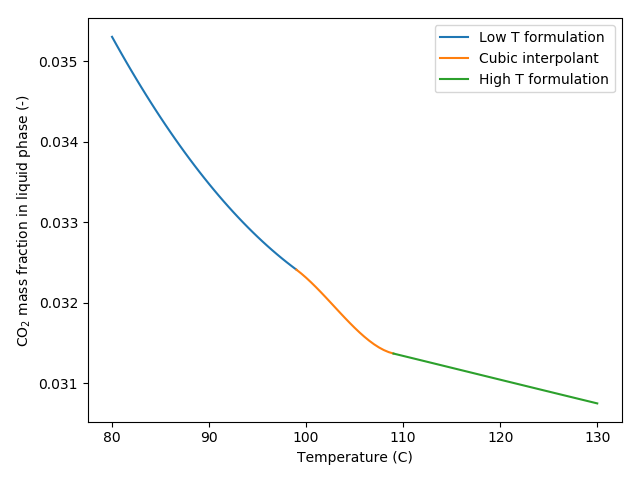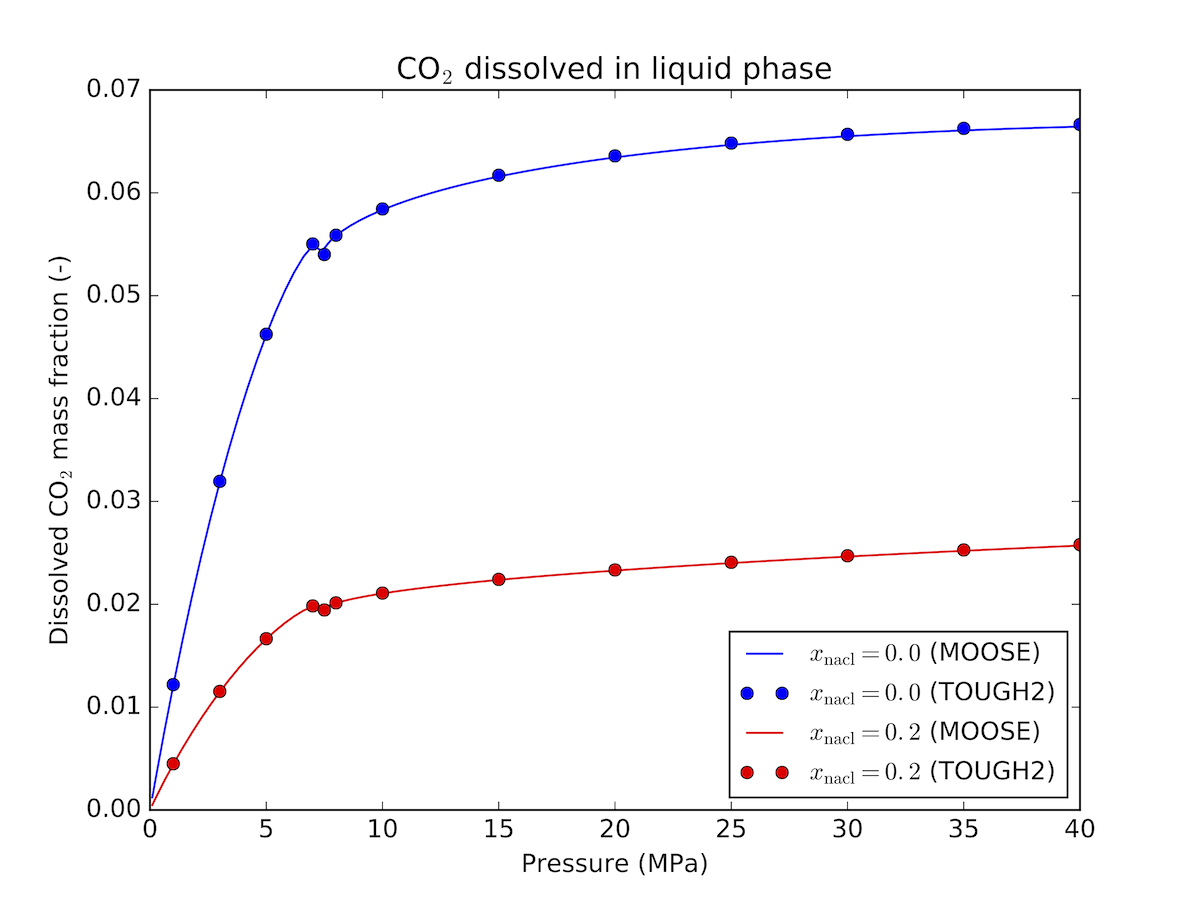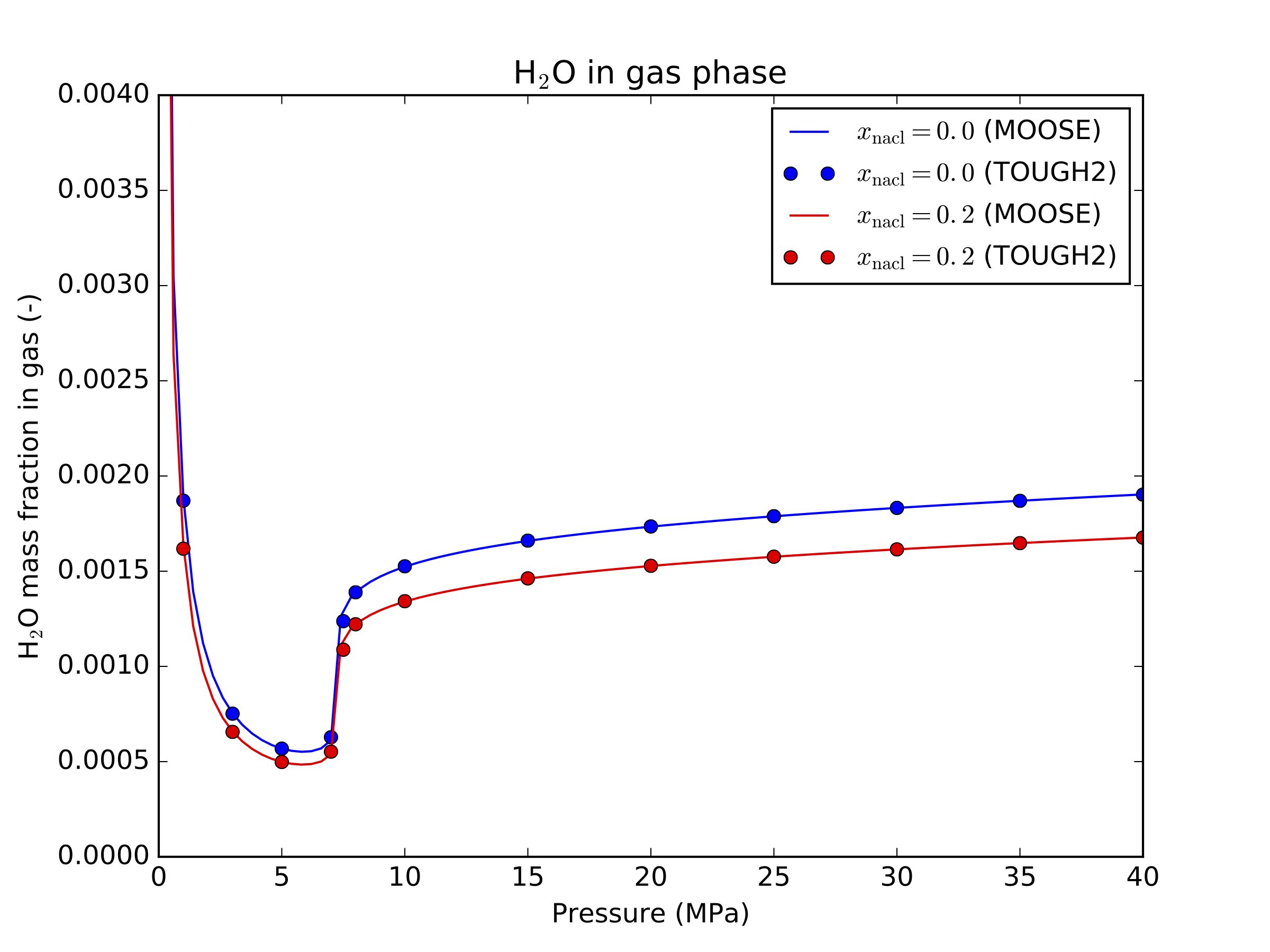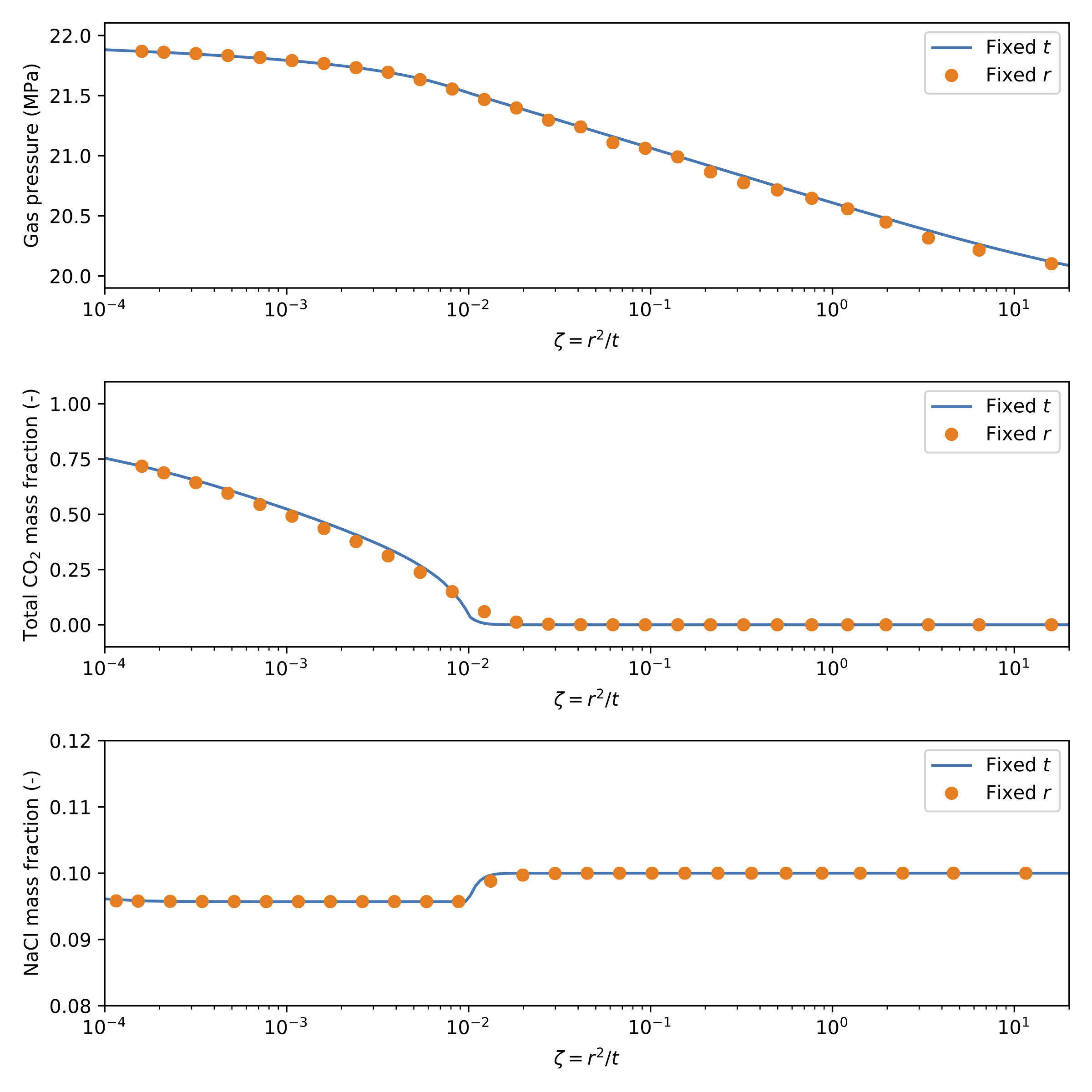# Brine and carbon dioxide

The brine-CO model available in PorousFlow is a high precision equation of state for brine and CO, including the mutual solubility of CO into the liquid brine and water into the CO-rich gas phase. This model is suitable for simulations of geological storage of CO in saline aquifers, and is valid for temperatures in the range and pressures less than 60 MPa.

Salt (NaCl) can be included as a nonlinear variable, allowing salt to be transported along with water to provide variable density brine.

## Phase composition

The mass fractions of CO in the liquid phase and HO in the gas phase are calculated using the accurate fugacity-based formulation of Spycher et al. (2003) and Spycher et al. (2005) for temperatures below 100C, and the elevated temperature formulation of Spycher and Pruess (2010) for temperatures above 110C.

As these formulations do not coincide for temperatures near 100C, a cubic polynomial is used to join the two curves smoothly, see Figure 1 for an example:Figure 1: Dissolved CO mass fraction in brine versus temperature showing the low and high temperature formulations joined smoothly by a cubic polynomial. Results for pressure of 10 MPa and salt mass fraction of 0.01

note

The mutual solubilities in the elevated temperature regime must be calculated iteratively, so an increase in computational expense can be expected.

This is similar to the formulation provided in the ECO2N module of TOUGH2 (Pruess et al., 1999), see Figure 2 and Figure 3 for a comparison between the two codes.Figure 2: Dissolved CO mass fraction in brine - comparison between MOOSE and TOUGH2 at 30CFigure 3: HO mass fraction in gas - comparison between MOOSE and TOUGH2 at 30C

As with many reservoir simulators, it is assumed that the phases are in instantaneous equilibrium, meaning that dissolution of CO into the brine and evaporation of water vapor into the CO phase happens instantaneously.

If the amount of CO present is not sufficient to saturate the brine, then no gas phase will be formed, and the dissolved CO mass fraction will be smaller than its equilibrium value. Similarly, if the amount of water vapor present is smaller than its equilibrium value in the gas phase, then no liquid phase can be formed.

If there is sufficient CO present to saturate the brine, then both liquid and gas phases will be present. In this case, the mass fraction of CO dissolved in the brine is given by its equilibrium value (for the current pressure, temperature and salinity). Similarly, the mass fraction of water vapor in the gas phase will be given by its equilibrium value in this two phase region.

## Thermophysical properties

### Density

The density of the aqueous phase with the contribution of dissolved CO is calculated using (1) where is the density of brine (supplied using a BrineFluidProperties UserObject), is the mass fraction of CO dissolved in the aqueous phase, and is the partial density of dissolved CO (Garcia, 2001).

As water vapor is only ever a small component of the gas phase in the temperature and pressure ranges that this class is valid for, the density of the gas phase is assumed to be simply the density of CO at the given pressure and temperature, calculated using a CO2FluidProperties UserObject.

### Viscosity

No contribution to the viscosity of each phase due to the presence of CO in the aqueous phase or water vapor in the gas phase is included. As a result, the viscosity of the aqueous phase is simply the viscosity of brine, while the viscosity of the gas phase is the viscosity of CO.

### Enthalpy

The enthalpy of the gas phase is simply the enthalpy of CO at the given pressure and temperature values, with no contribution due to the presence of water vapor included.

The enthalpy of the liquid phase is also calculated as a weighted sum of the enthalpies of each individual component, but also includes a contribution due to the enthalpy of dissolution (a change in enthalpy due to dissolution of the NCG into the water phase) (2) where is the enthalpy of brine, and is the enthalpy of CO in the liquid phase, which includes the enthalpy of dissolution (3)

In the range of pressures and temperatures considered, CO may exist as a gas or a supercritical fluid. Using a linear fit to the model of Duan and Sun (2003), the enthalpy of dissolution of both gas phase and supercritical CO is calculated as (4)

The calculation of brine and CO fluid properties can take a significant proportion of each simulation, so it is suggested that tabulated versions of both CO and brine proeprties are used using TabulatedFluidProperties.

## Implementation

### Variables

This class requires pressure of the gas phase, the total mass fraction of CO summed over all phases (see compositional flash for details), and the mass fraction of salt in the brine.

note

These variables must be listed as PorousFlow variables in the PorousFlowDictator UserObject

In the general case, three variables (gas porepressure, total CO mass fraction and salt mass fraction) are provided. As a result, the number of components in the PorousFlowDictator must be set equal to three.

[./dictator]
type = PorousFlowDictator
porous_flow_vars = 'pgas zi xnacl'
number_fluid_phases = 2
number_fluid_components = 3
[../]

(modules/porous_flow/test/tests/fluidstate/theis_brineco2.i)

The salt mass fraction can be treated as a constant, in which case only gas porepressure and total CO mass fraction are required, and the number of fluid components is two.

[./dictator]
type = PorousFlowDictator
porous_flow_vars = 'pgas z'
number_fluid_phases = 2
number_fluid_components = 2
[../]

(modules/porous_flow/test/tests/fluidstate/brineco2.i)

### UserObjects

This fluid state is implemented in the PorousFlowBrineCO2 UserObject. This UserObject is a GeneralUserObject that contains methods that provide a complete thermophysical description of the model given pressure, temperature and salt mass fraction.

[./fs]
type = PorousFlowBrineCO2
brine_fp = brine
co2_fp = co2
capillary_pressure = pc
[../]

(modules/porous_flow/test/tests/fluidstate/brineco2.i)

### Materials

The PorousFlowFluidState material provides all phase pressures, saturation, densities, viscosities etc, as well as all mass fractions of all fluid components in all fluid phases in a single material using the formulation provided in the PorousFlowBrineCO2 UserObject.

[./brineco2]
type = PorousFlowFluidState
gas_porepressure = pgas
z = z
temperature_unit = Celsius
xnacl = xnacl
capillary_pressure = pc
fluid_state = fs
[../]

(modules/porous_flow/test/tests/fluidstate/brineco2.i)

## Initial condition

The nonlinear variable representing CO is the total mass fraction of CO summed over all phases. In some cases, it may be preferred to provide an initial CO saturation, rather than total mass fraction. To allow an initial saturation to be specified, the PorousFlowFluidStateIC initial condition is provided. This initial condition calculates the total mass fraction of CO summed over all phases for a given saturation.

[ICs]
[./z]
type = PorousFlowFluidStateIC
saturation = 0.5
gas_porepressure = pgas
temperature = 50
variable = z
xnacl = 0.1
fluid_state = fs
[../]
[]

(modules/porous_flow/test/tests/fluidstate/brineco2_ic.i)

## Example

### 1D Radial injection

Injection into a one dimensional radial reservoir is a useful test of this class, as it admits a similarity solution , where is the radial distance from the injection well, and is time. This similarity solution holds even when complicated physics such as mutual solubility is included.

An example of a 1D radial injection problem is included in the test suite.

# Two phase Theis problem: Flow from single source.
# Constant rate injection 2 kg/s
# 1D cylindrical mesh
# Initially, system has only a liquid phase, until enough gas is injected
# to form a gas phase, in which case the system becomes two phase.
#
# This test takes a few minutes to run, so is marked heavy

[Mesh]
type = GeneratedMesh
dim = 1
nx = 2000
xmax = 2000
[]

[Problem]
type = FEProblem
coord_type = RZ
rz_coord_axis = Y
[]

[GlobalParams]
PorousFlowDictator = dictator
gravity = '0 0 0'
[]

[AuxVariables]
[./saturation_gas]
order = CONSTANT
family = MONOMIAL
[../]
[./x1]
order = CONSTANT
family = MONOMIAL
[../]
[./y0]
order = CONSTANT
family = MONOMIAL
[../]
[]

[AuxKernels]
[./saturation_gas]
type = PorousFlowPropertyAux
variable = saturation_gas
property = saturation
phase = 1
execute_on = timestep_end
[../]
[./x1]
type = PorousFlowPropertyAux
variable = x1
property = mass_fraction
phase = 0
fluid_component = 1
execute_on = timestep_end
[../]
[./y0]
type = PorousFlowPropertyAux
variable = y0
property = mass_fraction
phase = 1
fluid_component = 0
execute_on = timestep_end
[../]
[]

[Variables]
[./pgas]
initial_condition = 20e6
[../]
[./zi]
initial_condition = 0
[../]
[./xnacl]
initial_condition = 0.1
[../]
[]

[Kernels]
[./mass0]
type = PorousFlowMassTimeDerivative
fluid_component = 0
variable = pgas
[../]
[./flux0]
type = PorousFlowAdvectiveFlux
fluid_component = 0
variable = pgas
[../]
[./mass1]
type = PorousFlowMassTimeDerivative
fluid_component = 1
variable = zi
[../]
[./flux1]
type = PorousFlowAdvectiveFlux
fluid_component = 1
variable = zi
[../]
[./mass2]
type = PorousFlowMassTimeDerivative
fluid_component = 2
variable = xnacl
[../]
[./flux2]
type = PorousFlowAdvectiveFlux
fluid_component = 2
variable = xnacl
[../]
[]

[UserObjects]
[./dictator]
type = PorousFlowDictator
porous_flow_vars = 'pgas zi xnacl'
number_fluid_phases = 2
number_fluid_components = 3
[../]
[./pc]
type = PorousFlowCapillaryPressureConst
pc = 0
[../]
[./fs]
type = PorousFlowBrineCO2
brine_fp = brine
co2_fp = co2
capillary_pressure = pc
[../]
[]

[Modules]
[./FluidProperties]
[./co2sw]
type = CO2FluidProperties
[../]
[./co2]
type = TabulatedFluidProperties
fp = co2sw
[../]
[./water]
type = Water97FluidProperties
[../]
[./watertab]
type = TabulatedFluidProperties
fp = water
temperature_min = 273.15
temperature_max = 573.15
fluid_property_file = water_fluid_properties.csv
save_file = false
[../]
[./brine]
type = BrineFluidProperties
water_fp = watertab
[../]
[../]
[]

[Materials]
[./temperature]
type = PorousFlowTemperature
[../]
[./brineco2]
type = PorousFlowFluidState
gas_porepressure = pgas
z = zi
temperature_unit = Celsius
xnacl = xnacl
capillary_pressure = pc
fluid_state = fs
[../]
[./porosity]
type = PorousFlowPorosityConst
porosity = 0.2
[../]
[./permeability]
type = PorousFlowPermeabilityConst
permeability = '1e-12 0 0 0 1e-12 0 0 0 1e-12'
[../]
[./relperm_water]
type = PorousFlowRelativePermeabilityCorey
n = 2
phase = 0
s_res = 0.1
sum_s_res = 0.1
[../]
[./relperm_gas]
type = PorousFlowRelativePermeabilityCorey
n = 2
phase = 1
[../]
[]

[BCs]
[./rightwater]
type = DirichletBC
boundary = right
value = 20e6
variable = pgas
[../]
[]

[DiracKernels]
[./source]
type = PorousFlowSquarePulsePointSource
point = '0 0 0'
mass_flux = 2
variable = zi
[../]
[]

[Preconditioning]
[./smp]
type = SMP
full = true
[../]
[]

[Executioner]
type = Transient
solve_type = NEWTON
end_time = 1e5
[./TimeStepper]
type = IterationAdaptiveDT
dt = 1
growth_factor = 1.5
[../]
[]

[VectorPostprocessors]
[./line]
type = LineValueSampler
sort_by = x
start_point = '0 0 0'
end_point = '2000 0 0'
num_points = 10000
variable = 'pgas zi xnacl x1 saturation_gas'
execute_on = 'timestep_end'
[../]
[]

[Postprocessors]
[./pgas]
type = PointValue
point = '4 0 0'
variable = pgas
[../]
[./sgas]
type = PointValue
point = '4 0 0'
variable = saturation_gas
[../]
[./zi]
type = PointValue
point = '4 0 0'
variable = zi
[../]
[./massgas]
type = PorousFlowFluidMass
fluid_component = 1
[../]
[./x1]
type = PointValue
point = '4 0 0'
variable = x1
[../]
[./y0]
type = PointValue
point = '4 0 0'
variable = y0
[../]
[./xnacl]
type = PointValue
point = '4 0 0'
variable = xnacl
[../]
[]

[Outputs]
print_linear_residuals = false
perf_graph = true
[./csvout]
type = CSV
execute_on = timestep_end
execute_vector_postprocessors_on = final
[../]
[]

(modules/porous_flow/test/tests/fluidstate/theis_brineco2.i)

Initially, all of the CO injected is dissolved in the resident brine, as the amount of CO is less than the dissolved mass fraction at equilibrium. After a while, the brine near the injection well becomes saturated with CO, and a gas phase forms. This gas phase then spreads radially as injection continues. The mass fraction of salt in the brine decreases slightly where CO is present, due to the increased density of the brine following dissolution of CO.

A comparison of the results expressed in terms of the similarity solution is presented in Figure 4 for all three nonlinear variables for the case where is fixed (evaluated using Postprocessors), and also the case where is fixed (evaluated using a VectorPostprocessor).Figure 4: Similarity solution for 1D radial injection example

As these results show, the similarity solution holds for this problem.

1. Z. Duan and R. Sun. An improved model calculating CO$_2$ solubility in pure water and aqueous NaCl solutions from 273 to 533 K and from 0 to 2000 bar. Chemical geology, 193(3-4):257–271, 2003.[BibTeX]
2. J. E. Garcia. Density of aqueous solutions of CO$_2$. Technical Report, LBNL, 2001.[BibTeX]
3. K. Pruess, C. Oldenburg, and G. Moridis. TOUGH2 User's Guide, Version 2.0. Technical Report LBNL-43134, Lawrence Berkeley National Laboratory, Berkeley CA, USA, 1999.[BibTeX]
4. N. Spycher and K. Pruess. A phase-partitioning model for CO$_2$-brine mixtures at elevated temperatures and pressures: application to CO$_2$-enhanced geothermal systems. Transport in porous media, 82(1):173–196, 2010.[BibTeX]
5. N. Spycher, K. Pruess, and J. Ennis-King. CO$_2$-H$_2$O mixtures in the geological sequestration of CO$_2$. I. Assessment and calculation of mutual solubilities from 12 to 100C and up to 600 bar. Geochimica et Cosmochimica Acta, 67:3015–3031, 2003.[BibTeX]
6. N. Spycher, K. Pruess, and J. Ennis-King. CO$_2$-H$_2$O mixtures in the geological sequestration of CO$_2$. II. Partitioning in chloride brine at 12-100C and up to 600 bar. Geochimica et Cosmochimica Acta, 69:3309–3320, 2005.[BibTeX]